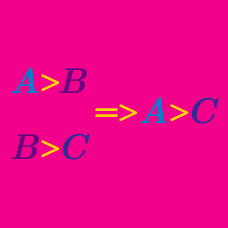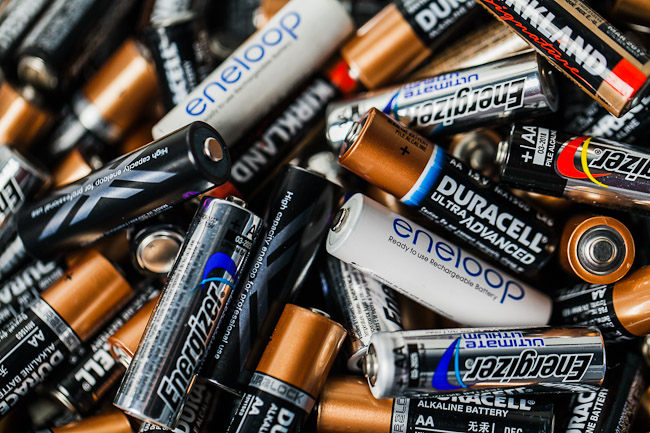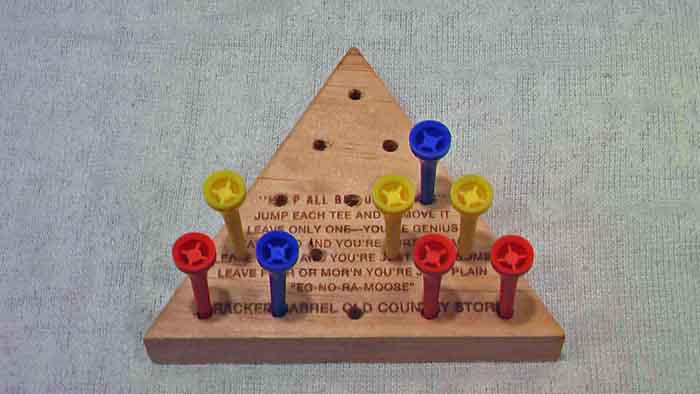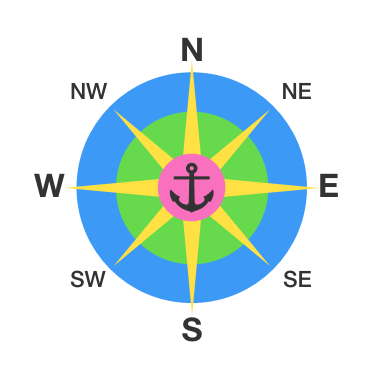Logic

# Logical Reasoning: Level 4 ChallengesYou go into your garage and find a pile of 100 batteries (all the same type).

You happen to know that half of them are good and half are bad, but you can't tell which is which.

You have a flashlight which uses two batteries, and requires both to work in order to turn on.

In the worst case scenario, with an optimal strategy, what is the minimum number of times you will need to put batteries into the flashlight before you can guarantee to get a working pair in the flashlight?


Details and Assumptions:

• The flashlight either turns on or it doesn't, i.e. there is no way to distinguish between one of the two working and neither one working.

• Your answer should be the number of "flashlight loadings" you will need to actually get your flashlight working, not just how many you would need to identify two good batteries.

###### Image credit: https://www.slrlounge.comJoe is playing a game involving the above board. He places 14 pegs on the board, leaving a single space empty. He then jumps a peg with an adjacent peg. The peg that has been jumped is removed.

For example, if Joe jumped the bottom-left yellow peg with the bottom-left red peg, then he would need to remove the yellow peg and move the red one to the space above the blue, on the third row.

The game ends when no more moves are possible.

What is the maximum amount of pegs that can be left when the game is over?

Note: I am not asking what the maximum possible number of pegs without any possible moves is--you must be able to get to the position by playing the game.

There is a king who wants to throw a party in 48 hours and he wants to serve a total of 500 barrels of wine at the party. Unfortunately, he knows that one of the barrels has been poisoned and he does not know which one. When consumed, the poison takes between 23 and 24 hours to kill.

In order to determine which barrel the poison is in, the king wants to use his prisoners as taste testers. What is the fewest number of prisoners that he needs to test the barrels in 48 hours?

Note: The king doesn't care about the number of prisoners that die. He just wants to minimize the number of prisoners used.There is a diamond hidden on an $N\times N$ grid at location $(x_D,y_D)$, where $x_D$ and $y_D$ are integers.

Every guess, you suggest a pair of coordinates ($x_G$, $y_G$). And, if you get it wrong you are given a hint as to where to go to continue looking. You are told either NW, N, NE, E, SE, S, SW, or W:

• W implies $x_D < x_G$ and $y_D = y_G$
• NW implies $x_D < x_G$ and $y_D > y_G$
• etc.

If you can get the diamond with 10 guesses or less (i.e. at most 9 wrong guesses and one right one), you get to keep the diamond. What is the largest $N$ for which you can guarantee success?There is a circle of $n$ light bulbs with a switch next to each of them. Each switch can be flipped between two positions, thereby toggling the on/off states of three lights: its own and the two lights adjacent to it. Initially, all the lights are off.

Let the minimum number of flips needed to turn on all the $n=12$ and $n=13$ light bulbs be $a$ and $b$, respectively. Then what is the value of $a+b$?

×